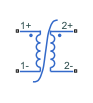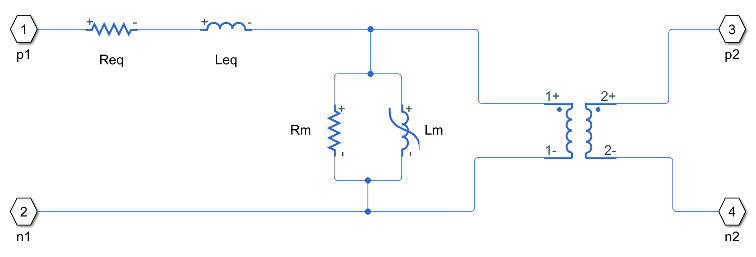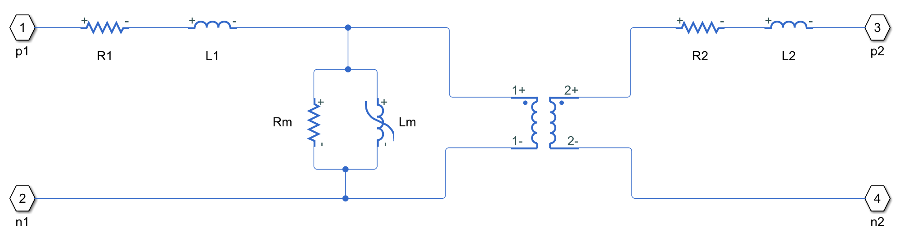# Nonlinear Transformer

Transformer with nonideal core

•Libraries:
Simscape / Electrical / Passive / Transformers

## Description

The Nonlinear Transformer block represents a transformer with a nonideal core. A core may be nonideal due to its magnetic properties and dimensions. The equivalent circuit topology depends upon which of the two winding leakage parameterization options you select:

• `Combined primary and secondary values`• `Separate primary and secondary values`where:

• Req is the combined winding resistance.

• Leq is the combined leakage inductance.

• R1 is the primary winding resistance.

• L1 is the primary winding inductance.

• R2 is the secondary winding resistance.

• L2 is the secondary winding inductance.

• Rm is the magnetization resistance.

• Lm is the magnetization inductance.

The block provides the following parameterization options for the nonlinear magnetization inductance:

• `Single inductance (linear)`

• `Single saturation point`

• `Magnetic flux versus current characteristic`

• ```Magnetic flux density versus magnetic field strength characteristic```

• ```Magnetic flux density versus magnetic field strength characteristic with hysteresis```

## Ports

### Conserving

expand all

Electrical conserving port associated with the primary winding positive polarity.

Electrical conserving port associated with the primary winding negative polarity.

Electrical conserving port associated with the primary winding negative polarity.

Electrical conserving port associated with the secondary winding positive polarity.

Electrical conserving port associated with the secondary winding negative polarity.

## Parameters

expand all

### Main

The number of turns of wire on the primary winding of the transformer.

The number of turns of wire on the secondary winding of the transformer.

Select one of the following methods for the winding leakage parameterization:

• `Combined primary and secondary values` — Use the lumped resistance and inductance values representing the combined leakage in the primary and secondary windings. This is the default option.

• `Separate primary and secondary values` — Use separate resistances and inductances to represent leakages in the primary and secondary windings.

The lumped equivalent resistance Req, which represents the combined power loss of the primary and secondary windings.

#### Dependencies

This parameter is visible only when you select ```Combined primary and secondary values``` for the Winding parameterized by parameter.

The lumped equivalent inductance Leq, which represents the combined magnetic flux loss of the primary and secondary windings.

#### Dependencies

This parameter is visible only when you select ```Combined primary and secondary values``` for the Winding parameterized by parameter.

The resistance R1, which represents the power loss of the primary winding.

#### Dependencies

This parameter is visible only when you select ```Separate primary and secondary values``` for the Winding parameterized by parameter.

The inductance L1, which represents the magnetic flux loss of the primary winding.

#### Dependencies

This parameter is visible only when you select ```Separate primary and secondary values``` for the Winding parameterized by parameter.

The resistance R2, which represents the power loss of the secondary winding.

#### Dependencies

This parameter is visible only when you select ```Separate primary and secondary values``` for the Winding parameterized by parameter.

The inductance L2, which represents the magnetic flux loss of the secondary winding.

#### Dependencies

This parameter is visible only when you select ```Separate primary and secondary values``` for the Winding parameterized by parameter.

Averaging period for power logging

### Magnetization

The resistance Rm, which represents the magnetic losses in the transformer core.

Select one of the following methods for the nonlinear magnetization inductance parameterization:

• `Single inductance (linear)` — Provide the unsaturated inductance value.

• `Single saturation point` — Provide the values for the unsaturated and saturated inductances, as well as saturation magnetic flux. This is the default option.

• `Magnetic flux versus current characteristic` — Provide the current vector and the magnetic flux vector, to populate the magnetic flux versus current lookup table.

• ```Magnetic flux density versus field strength characteristic``` — Provide the values for effective core length and cross-sectional area, as well as the magnetic field strength vector and the magnetic flux density vector, to populate the magnetic flux density versus magnetic field strength lookup table.

• ```Magnetic flux density versus field strength characteristic with hysteresis``` — In addition to the number of turns and the effective core length and cross-sectional area, provide the values for the initial anhysteretic B-H curve gradient, the magnetic flux density and field strength at a certain point on the B-H curve, as well as the coefficient for the reversible magnetization, bulk coupling coefficient, and inter-domain coupling factor, to define magnetic flux density as a function of both the current value and the history of the field strength.

The value of inductance used when the magnetization inductance Lm is operating in its linear region.

#### Dependencies

This parameter is visible only when you select ```Single inductance (linear)``` or `Single saturation point` for the Magnetization inductance parameterized by parameter.

The value of inductance used when the magnetization inductance Lm is operating beyond its saturation point.

#### Dependencies

This parameter is visible only when you select ```Single saturation point``` for the Magnetization inductance parameterized by parameter.

The value of magnetic flux at which the magnetization inductance Lm saturates.

#### Dependencies

This parameter is visible only when you select ```Single saturation point``` for the Magnetization inductance parameterized by parameter.

The current data used to populate the magnetic flux versus current lookup table.

#### Dependencies

This parameter is visible only when you select ```Magnetic flux versus current characteristic``` for the Magnetization inductance parameterized by parameter.

The magnetic flux data used to populate the magnetic flux versus current lookup table.

#### Dependencies

This parameter is visible only when you select ```Magnetic flux versus current characteristic``` for the Magnetization inductance parameterized by parameter.

The magnetic field strength data used to populate the magnetic flux density versus magnetic field strength lookup table.

#### Dependencies

This parameter is visible only when you select ```Magnetic flux density versus field strength characteristic``` for the Magnetization inductance parameterized by parameter.

The magnetic flux density data used to populate the magnetic flux density versus magnetic field strength lookup table.

#### Dependencies

This parameter is visible only when you select ```Magnetic flux density versus field strength characteristic``` for the Magnetization inductance parameterized by parameter.

The effective core length, that is, the average distance of the magnetic path around the transformer core.

#### Dependencies

This parameter is visible only when you select ```Magnetic flux density versus field strength characteristic``` for the Magnetization inductance parameterized by parameter.

The effective core cross-sectional area, that is, the average area of the magnetic path around the transformer core.

#### Dependencies

This parameter is visible only when you select ```Magnetic flux density versus field strength characteristic``` for the Magnetization inductance parameterized by parameter.

The gradient of the anhysteretic (no hysteresis) B-H curve around zero field strength. Set it to the average gradient of the positive-going and negative-going hysteresis curves.

#### Dependencies

This parameter is visible only when you select ```Magnetic flux density versus field strength characteristic with hysteresis``` for the Magnetization inductance parameterized by parameter.

Specify a point on the anhysteretic curve by providing its flux density value. Picking a point at high field strength where the positive-going and negative-going hysteresis curves align is the most accurate option.

#### Dependencies

This parameter is visible only when you select ```Magnetic flux density versus field strength characteristic with hysteresis``` for the Magnetization inductance parameterized by parameter.

The corresponding field strength for the point that you define by the Flux density point on anhysteretic B-H curve parameter.

#### Dependencies

This parameter is visible only when you select ```Magnetic flux density versus field strength characteristic with hysteresis``` for the Magnetization inductance parameterized by parameter.

The proportion of the magnetization that is reversible. The value should be greater than zero and less than one.

#### Dependencies

This parameter is visible only when you select ```Magnetic flux density versus field strength characteristic with hysteresis``` for the Magnetization inductance parameterized by parameter.

The Jiles-Atherton parameter that primarily controls the field strength magnitude at which the B-H curve crosses the zero flux density line.

#### Dependencies

This parameter is visible only when you select ```Magnetic flux density versus field strength characteristic with hysteresis``` for the Magnetization inductance parameterized by parameter.

The Jiles-Atherton parameter that primarily affects the points at which the B-H curves intersect the zero field strength line. Typical values are in the range of 1e-4 to 1e-3.

#### Dependencies

This parameter is visible only when you select ```Magnetic flux density versus field strength characteristic with hysteresis``` for the Magnetization inductance parameterized by parameter.

The lookup table interpolation option. Select one of the following interpolation methods:

• `Linear` — Select this option to get the best performance.

• `Smooth` — Select this option to produce a continuous curve with continuous first-order derivatives.

For more information on interpolation algorithms, see the PS Lookup Table (1D) block reference page.

#### Dependencies

This parameter is visible only when you select ```Magnetic flux versus current characteristic``` or ```Magnetic flux density versus field strength characteristic``` for the Magnetization inductance parameterized by parameter.

### Initial Conditions

The value of current through the combined leakage inductance Leq at time zero.

#### Dependencies

This parameter is visible only when you select ```Combined primary and secondary values``` for the Winding parameterized by parameter on the Main tab.

The value of current through the primary leakage inductance L1 at time zero.

#### Dependencies

This parameter is visible only when you select ```Separate primary and secondary values``` for the Winding parameterized by parameter on the Main tab.

The value of current through the secondary leakage inductance L2 at time zero.

#### Dependencies

This parameter is visible only when you select ```Separate primary and secondary values``` for the Winding parameterized by parameter on the Main tab.

Select the appropriate initial state specification option:

• `Current` — Specify the initial state of the magnetization inductance Lm by the initial current. This is the default option.

• `Magnetic flux` — Specify the initial state of the magnetization inductance Lm by the magnetic flux.

#### Dependencies

This parameter is not visible only when you select ```Magnetic flux density versus magnetic field strength characteristic with hysteresis``` for the Magnetization inductance parameterized by parameter on the Magnetization tab.

The initial current value used to calculate the value of magnetic flux within the magnetization inductance Lm at time zero. This is the current passing through the magnetization inductance Lm. Total magnetization current consists of current passing through the magnetization resistance Rm and current passing through the magnetization inductance Lm.

#### Dependencies

This parameter is visible only when you select `Current` for the Specify magnetization inductance initial state by parameter.

The value of the magnetic flux in the magnetization inductance Lm at time zero.

#### Dependencies

This parameter is visible only when you select ```Magnetic flux``` for the Specify magnetization inductance initial state by parameter.

The value of magnetic flux density at time zero. This parameter is visible only when you select ```Magnetic flux density versus magnetic field strength characteristic with hysteresis``` for the Magnetization inductance parameterized by parameter on the Magnetization tab.

#### Dependencies

This parameter is visible only when you select ```Magnetic flux density versus field strength characteristic with hysteresis``` for the Magnetization inductance parameterized by parameter.

The value of magnetic field strength at time zero.

#### Dependencies

This parameter is visible only when you select ```Magnetic flux density versus field strength characteristic with hysteresis``` for the Magnetization inductance parameterized by parameter.

### Parasitics

Use this parameter to represent small parasitic effects in parallel to the combined leakage inductance Leq. A small parallel conductance may be required for the simulation of some circuit topologies.

#### Dependencies

This parameter is visible only when you select ```Combined primary and secondary values``` for the Winding parameterized by parameter on the Main tab.

Use this parameter to represent small parasitic effects in parallel to the primary leakage inductance L1. A small parallel conductance may be required for the simulation of some circuit topologies.

#### Dependencies

This parameter is visible only when you select ```Separate primary and secondary values``` for the Winding parameterized by parameter on the Main tab.

Use this parameter to represent small parasitic effects in parallel to the secondary leakage inductance L2. A small parallel conductance may be required for the simulation of some circuit topologies.

#### Dependencies

This parameter is visible only when you select ```Separate primary and secondary values``` for the Winding parameterized by parameter on the Main tab.

## Version History

Introduced in R2012b